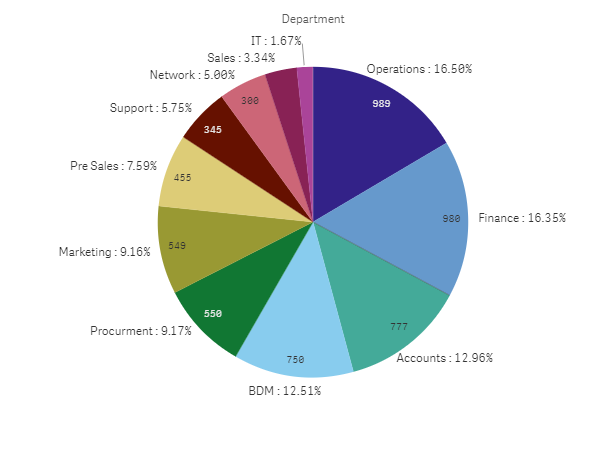# New to Qlik Sense

Discussion board where members can get started with Qlik Sense.

Highlighted
Valued Contributor III

## double values

Hi all,

is there any way to show both values and %  at a time on pie chart Qliskense?

anyone has some sample please share

Tags (1)
1 Solution

Accepted SolutionsPartner

## Re: double values

the

sum(YourMeasure) = count({<JDate={">=\$(=vStartDate)<=\$(=vEndDate)"},[JStatus]={'Joined'}>}[ID])

maybe it's a syntax thing: let's try again:

dimension:

=[In/Ex]&' : '& aggr(num(count({<JDate={">=\$(=vStartDate)<=\$(=vEndDate)"},[JStatus]={'Joined'}>}[ID])

/count( total {<[In/Ex]=,JDate={">=\$(=vStartDate)<=\$(=vEndDate)"},[JStatus]={'Joined'}>}[ID]),'#,##0.00%'),[In/Ex])

measure:

count({<JDate={">=\$(=vStartDate)<=\$(=vEndDate)"},[JStatus]={'Joined'}>}[ID])

10 RepliesPartner

## Re: double values

sure:

in u pie chart;

as dimension:

=YourDim&' : '& aggr(num(sum(YourMeasure)/sum(total{<YourDim>} YourMeasure),'#,##0.00%'),YourDim)

as a measure:

sum(YourMeasure)

Under aspect > presentation, show the values:result (per aalogy):Valued Contributor III

## Re: double values

thanks.

but in my case its not working  below am using  what is the issue here?

Dim: [In/Ex]

Meausre:count({<JDate={">=\$(=vStartDate)<=\$(=vEndDate)"},[JStatus]={'Joined'}>}[ID])

=[In/Ex]' : '& aggr(num(sum(count({<JDate={">=\$(=vStartDate)<=\$(=vEndDate)"},[JStatus]={'Joined'}>}[ID]))

/sum(total{<[In/Ex]>}

count({<JDate={">=\$(=vStartDate)<=\$(=vEndDate)"},

[JStatus]={'Join'}>}[ID])),'#,##0.00%'),[In/Ex])Partner

## Re: double values

Your dim would be :

=[In/Ex]' : '&

aggr(num( (count({<JDate={">=\$(=vStartDate)<=\$(=vEndDate)"},[JStatus]={'Joined'}>}[ID])

/

count(total {<[In/Ex]=, JDate={">=\$(=vStartDate)<=\$(=vEndDate)"},[JStatus]={'Joined'}>}[ID])),'#,##0.00%'),[In/Ex])

Valued Contributor III

## Re: double values

showing as invalid dimension

and what could be my measure?  can I add sum(my meausre) ?Partner

## Re: double values

the

sum(YourMeasure) = count({<JDate={">=\$(=vStartDate)<=\$(=vEndDate)"},[JStatus]={'Joined'}>}[ID])

maybe it's a syntax thing: let's try again:

dimension:

=[In/Ex]&' : '& aggr(num(count({<JDate={">=\$(=vStartDate)<=\$(=vEndDate)"},[JStatus]={'Joined'}>}[ID])

/count( total {<[In/Ex]=,JDate={">=\$(=vStartDate)<=\$(=vEndDate)"},[JStatus]={'Joined'}>}[ID]),'#,##0.00%'),[In/Ex])

measure:

count({<JDate={">=\$(=vStartDate)<=\$(=vEndDate)"},[JStatus]={'Joined'}>}[ID])

Valued Contributor III

## Re: double values

yes its working thanks a lotbut colour it  is showing grey only one colour . total pie. ?

I want two colursPartner

## Re: double values

under color, color by dimension and choose ur original dimension (not the modified one) but [In/Ex].

Valued Contributor III

## Re: double values

thanksomerben2016Partner

## Re: double values

it's omarbensalem